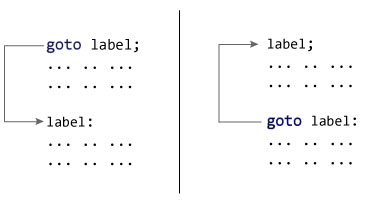# C++ goto Statement

In this article, you'll learn about goto statment, how it works and why should it be avoided.

In C++ programming, goto statement is used for altering the normal sequence of program execution by transferring control to some other part of the program.

## Syntax of goto Statement

```goto label;
... .. ...
... .. ...
... .. ...
label:
statement;
... .. ...```

In the syntax above, label is an identifier. When `goto label;` is encountered, the control of program jumps to `label:` and executes the code below it.Working of goto in C++

### Example: goto Statement

``````// This program calculates the average of numbers entered by the user.
// If the user enters a negative number, it ignores the number and
// calculates the average number entered before it.

# include <iostream>
using namespace std;

int main()
{
float num, average, sum = 0.0;
int i, n;

cout << "Maximum number of inputs: ";
cin >> n;

for(i = 1; i <= n; ++i)
{
cout << "Enter n" << i << ": ";
cin >> num;

if(num < 0.0)
{
// Control of the program move to jump:
goto jump;
}
sum += num;
}

jump:
average = sum / (i - 1);
cout << "\nAverage = " << average;
return 0;
}``````

Output

```Maximum number of inputs: 10
Enter n1: 2.3
Enter n2: 5.6
Enter n3: -5.6

Average = 3.95```

You can write any C++ program without the use of `goto` statement and is generally considered a good idea not to use them.

### Reason to Avoid goto Statement

The goto statement gives the power to jump to any part of a program but, makes the logic of the program complex and tangled.

In modern programming, the goto statement is considered a harmful construct and a bad programming practice.

The goto statement can be replaced in most of C++ program with the use of break and continue statements.

Did you find this article helpful?